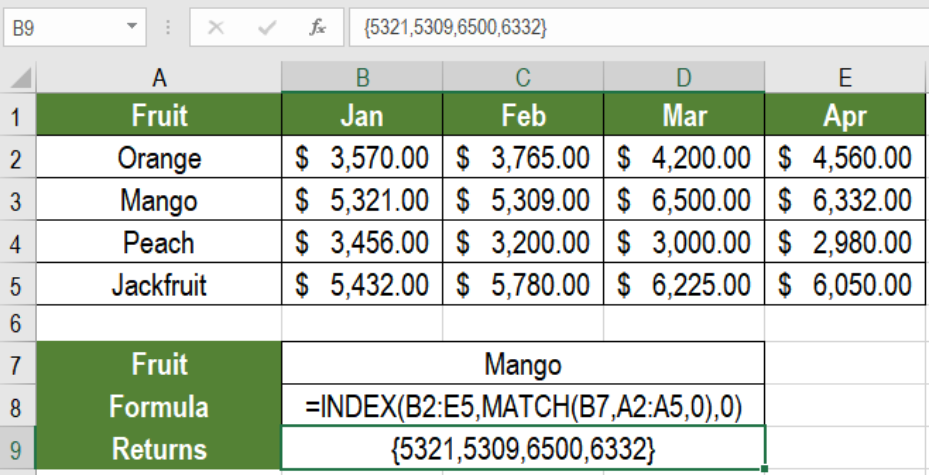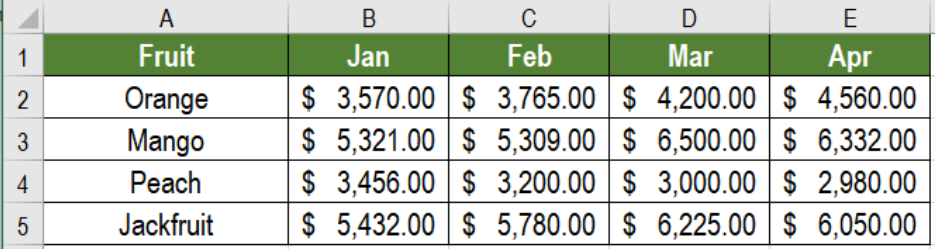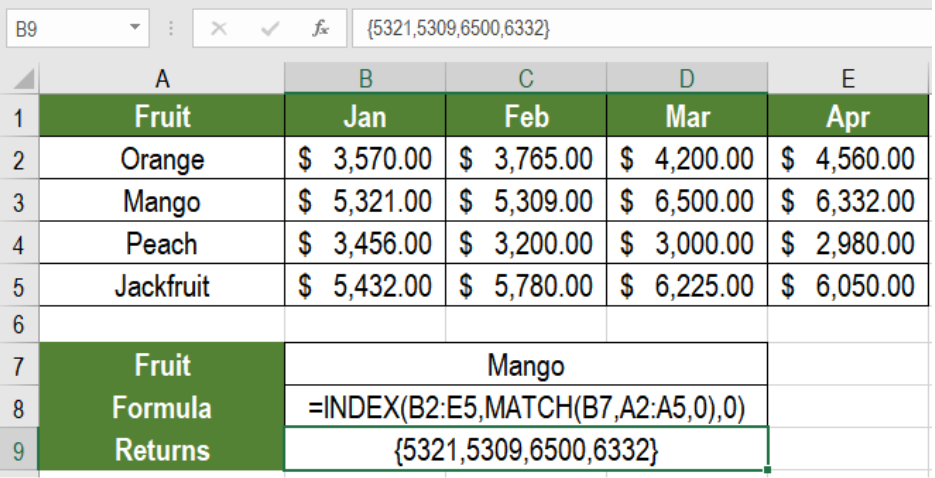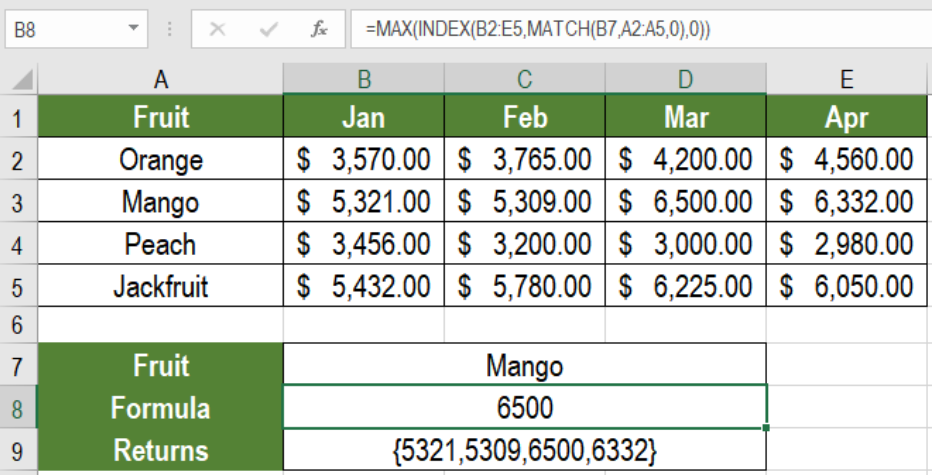Get instant live expert help with Excel or Google Sheets“My Excelchat expert helped me in less than 20 minutes, saving me what would have been 5 hours of work!”

#### Post your problem and you'll get expert help in seconds

Your message must be at least 40 characters
Our professional experts are available now. Your privacy is guaranteed.

# How to Lookup an Entire Row in Excel

Lookup is one of the mostly performed tasks in Microsoft Excel. We often require to perform lookup on different data. Sometimes, the result needs to be an entire row. The combination of the INDEX and MATCH functions allow us to lookup an entire row. In this tutorial, we will learn how to lookup an entire row in Excel.Figure 1. Example of How to Lookup an Entire Row in Excel

## Generic Formula

`=INDEX(data,MATCH(value,array,0),0)`

## How the Formula Works

Here, we use the MATCH function to get the row index. The lookup value is searched inside the range. We use an exact match here. Next, MATCH returns the row index. This goes into the INDEX function as the row number.

INDEX uses the previous range as the array, and the row index as the row number. We set the column number to be 0 Finally, INDEX returns the entire row as an array.

## Setting up Data

The following example uses a fruit sales database. Column A has the names, columns B to E has the sales from January to April.Figure 2. The Sample Data Set

To find out the sales information on oranges, we need to

• Go to cell B8.
• Assign the formula `=INDEX(B2:E6,MATCH(B7,A2:A6,0),0)` to B8.
• Press Enter.Figure 3. Applying the Formula

This will return the entire sales information of mangoes.

Excel does not display this information. However, we can use it with other functions like LARGE, MIN, MAX, SUM etc. To find out the highest sales for mangoes in B8, we can use the formula `=MAX(INDEX(B2:E5,MATCH(B7,A2:A5,0),0))`.Figure 4. Example of Using Other Functions with Entire Row Lookups

Generally, we can lookup a single cell only. However using the INDEX and MATCH formula helps us to perform a lookup on an entire row. This helps a lot to analyze data effective and efficiently.

Most of the time, the problem you will need to solve will be more complex than a simple application of a formula or function. If you want to save hours of research and frustration, try our live Excelchat service! Our Excel Experts are available 24/7 to answer any Excel question you may have. We guarantee a connection within 30 seconds and a customized solution within 20 minutes.

Are you still looking for help with Conditional Formatting? View our comprehensive round-up of Conditional Formatting tutorials here.

### Did this post not answer your question? Get a solution from connecting with the expert.Another blog reader asked this question today on Excelchat:## Subscribe to Excelchat.coAnother blog reader asked this question today on Excelchat: